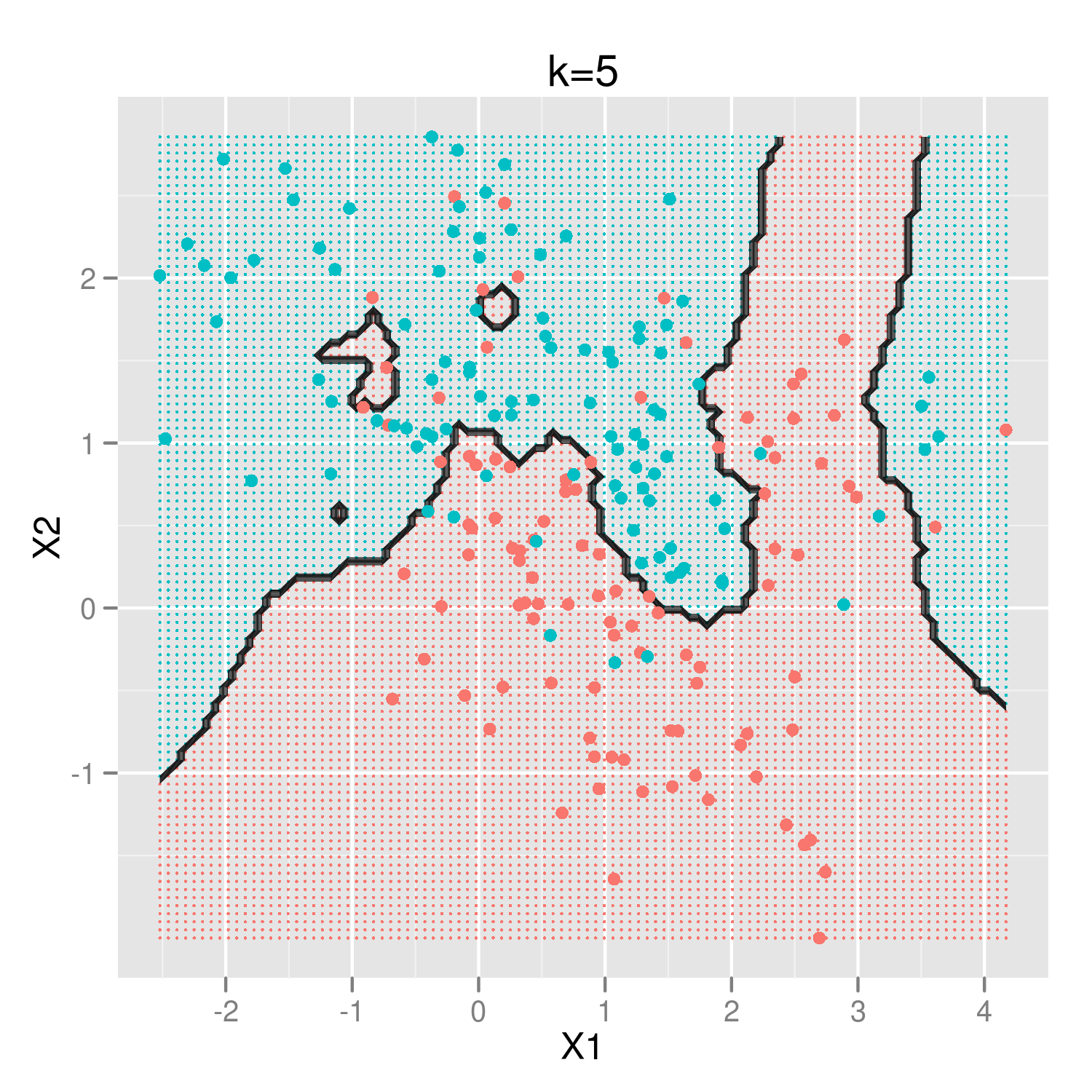I've recently started the hacker dojo class data mining 201 taught by Mike Bowles.

In week 1, we talked about the k nearest neighbors algorithms and the relationship between model complexity and parameter k.

## Load and Visualize Data

To illustrate let's use data provided from the book Elements of Statistical Learning by T. Hastie, R. Tibshirani, and J. Friedman available here.The above graph shows the original data, with the blue dots showing where Y=1 and the pink dots show where Y=0. If we are given a new point with an (X1, X2) input value, how can we predict Y?

K-nearest-neighbors ("knn") uses a very simple idea to determine unknown points. It looks at the k closest points, and if there are more blue points close-by, knn will predict that the new point is also blue. If there are more pink points close-by, knn will predict that the new point is pink. Easy.

The only input we provide is how many points, or neighbors, the algorithm should consider. This is the k value.

## Create Test Data

The above code block creates a grid of 10,000 points equally spaced in X1 and X2. We'll then overlay these grid points with the original points above, and then with different values of k, we'll see which group knn thinks each grid point should is in.## Try Different Values for k

The function knnplot takes our training data (the plot of pink and blue dots), the test data (the grid of points), the parameter k, and then predicts whether each of the grid points is blue or pink.

For example, if we call knnplot with a k=5, we get the following graph. The black line shows the decisions boundaries, and the coloring shows whether knn predicted the point to be pink or blue.We can see that the decision boundary is fairly complex. If we user a lower number of k, out decision boundary is even more complex, meaning that the solution is more complex. The image below shows the value for k=3, that is the algorithm only looks to the three closest points to determine the new points value.To decide on the optimal k, we'd carve out a subset of the original data and see how accurately the algorithm predicted these known values, and then pick k based on whichever value did best according to whatever criteria we choose.

## Visualize all possible values of k

To see how the decision boundary changes for many values of k, see the video below which animates all k values from 1 to 200. You should notice two things:

1. for even values of k, the decision boundary gets very kinky. That's because the algorithm breaks ties randomly. We can't have ties with an odd k, but you can with an even k.

2. as k gets larger, the decision boundary becomes less meangingful. Suppose k equaled the total number of inputs, in this case 200. That means knn is looking at all known points, and in our case we have an even split between the blue and pink points. Therefore knn will see that 100 neighbors are blue, 100 and pink, and then it'll randomly decide which group the new point belongs to. So k>=200 means we're just flipping a coin.

The code in the last chunk creates a png file for each value of k. We can glue all these png files together into an mp4 file using the Unix command line tool ffmpeg like so:

### R documentation on knn:

For each row of the test set, the k nearest (in Euclidean distance) training set vectors are found, and the classification is decided by majority vote, with ties broken at random. If there are ties for the kth nearest vector, all candidates are included in the vote.